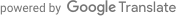## 數學筆記

$\tau_i = Y_i(1) - Y_i(0) \qquad(4.1)$

1 $$Y_1(1)$$ $$Y_1(0)$$ $$\tau_1$$
2 $$Y_2(1)$$ $$Y_2(0)$$ $$\tau_2$$
$$\vdots$$ $$\vdots$$ $$\vdots$$ $$\vdots$$
ñ $$Y_N(1)$$ $$Y_N(0)$$ $$\tau_N$$

$\text{ATE} = \frac{1}{N} \sum_{i=1}^N \tau_i \qquad(4.2)$

$\text{ATE} = \frac{1}{N} \sum_{i=1}^N Y_i(1) - \frac{1}{N} \sum_{i=1}^N Y_i(0) \qquad(4.3)$

$\widehat{\text{ATE}} = \underbrace{\frac{1}{N_t} \sum_{i:W_i=1} Y_i(1)}_{\text{average edits, treatment}} - \underbrace{\frac{1}{N_c} \sum_{i:W_i=0} Y_i(0)}_{\text{average edits, control}} \qquad(4.4)$

$Y_i(W_i, \mathbf{W_{-i}}) = Y_i(W_i) \quad \forall \quad \mathbf{W_{-i}} \qquad(4.5)$

$SE(\widehat{\text{ATE}}) = \sqrt{\frac{1}{N-1} \left(\frac{m \text{Var}(Y_i(0))}{N-m} + \frac{(N-m) \text{Var}(Y_i(1))}{m} + 2\text{Cov}(Y_i(0), Y_i(1)) \right)} \qquad(4.6)$

$\text{ATE}' = \frac{1}{N} \sum_{i=1}^N ((Y_i(1) - X_i) - (Y_i(0) - X_i)) \qquad(4.7)$

$SE(\widehat{\text{ATE}'}) = \sqrt{\frac{1}{N-1} \left( \text{Var}(Y_i(0) - X_i) + \text{Var}(Y_i(1) - X_i) + 2\text{Cov}(Y_i(0) - X_i, Y_i(1) - X_i) \right)} \qquad(4.8)$

$\frac{\text{Cov}(Y_i(0), X_i)}{\text{Var}(X_i)} + \frac{\text{Cov}(Y_i(1), X_i)}{\text{Var}(X_i)} > 1\qquad(4.9)$